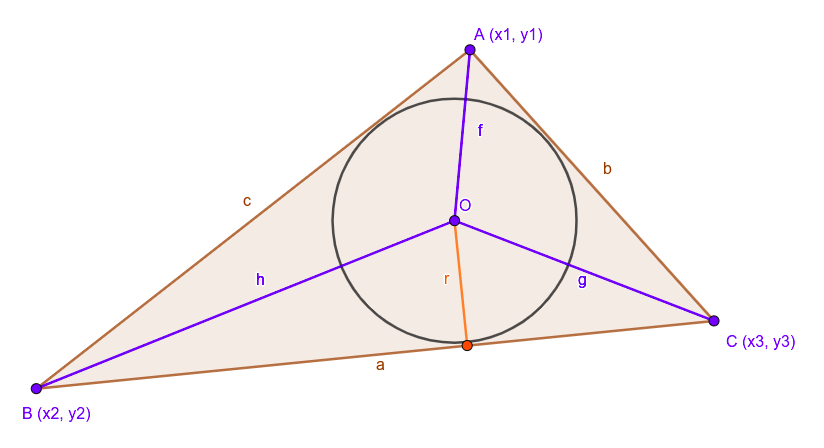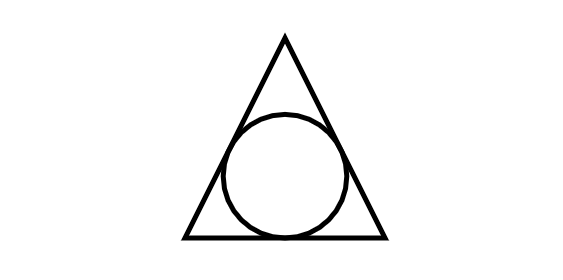# How to draw Inscribed circle of a triangle using p5.js ?

In this article, we will see how to draw inscribed circle (incircle) form a triangle. The incircle is nothing but the largest possible circle that can be drawn on the inside of a plane figure. So basically all sides (three) of triangle must be touched by the circle surface but not to cross the sidebars.

Mathematics Background: In given triangle ABC as shown below.• The center of the inscribed-circle (known as in-center) of a triangle is defined as:Implementation:

• Step 1: In Computer Graphics we represent a triangle by its angular points rather than its side length meaning the input would be the vertices of the triangle! So we need to convert that into the side-lengths a, b, c so that we could use it for the formula. To achieve this we use this simple getSides helper function:
Code:

 // Get Sides from Angular points  function getSides(Ax, Ay, Bx, By, Cx, Cy){    return {      a: dist(Bx, By, Cx, Cy),      b: dist(Cx, Cy, Ax, Ay),      c: dist(Ax, Ay, Bx, By),    }  }

• Step 2: Now, we can convert angular points to sides we can simply plug the formula to get the desired result. Here, the full P5.js sketch which draws the incircle of a triangle:

Code:

 function setup() {    createCanvas(640, 480);  }     function draw() {    background(255);    noFill()    stroke(0)    strokeWeight(5)    triangle(320, 140, 220, 340, 420, 340)    drawInCircle(320, 140, 220, 340, 420, 340)    noLoop()  }     function drawInCircle(x1, y1, x2, y2, x3, y3){    let side=getSides(x1, y1, x2, y2, x3, y3)    let a=side.a, b=side.b, c=side.c;    let inCenter=getIncenter(a, b, c, x1, y1, x2, y2, x3, y3);    let inRadius=getInradius(a, b, c);    circle(inCenter.x, inCenter.y, 2*inRadius)  }     // Helper Function: Get Sides from Angular points  function getSides(Ax, Ay, Bx, By, Cx, Cy){    return {      a: dist(Bx, By, Cx, Cy),      b: dist(Cx, Cy, Ax, Ay),      c: dist(Ax, Ay, Bx, By),    }  }  function getIncenter(a, b, c, x1, y1, x2, y2, x3, y3){    return {      x: (a*x1 + b*x2 + c*x3)/(a + b + c),      y: (a*y1 + b*y2 + c*y3)/(a + b + c)    }  }     function getInradius(a, b, c){    let s=(a+b+c)/2    // Semi-perimeter    let area=sqrt(s*(s-a)*(s-b)*(s-c))    return area/s  }

• Output:Note: You can edit and run your code by using online editor.My Personal Notes arrow_drop_upCheck out this Author's contributed articles.

If you like GeeksforGeeks and would like to contribute, you can also write an article using contribute.geeksforgeeks.org or mail your article to contribute@geeksforgeeks.org. See your article appearing on the GeeksforGeeks main page and help other Geeks.

Please Improve this article if you find anything incorrect by clicking on the "Improve Article" button below.

Be the First to upvote.

Please write to us at contribute@geeksforgeeks.org to report any issue with the above content.